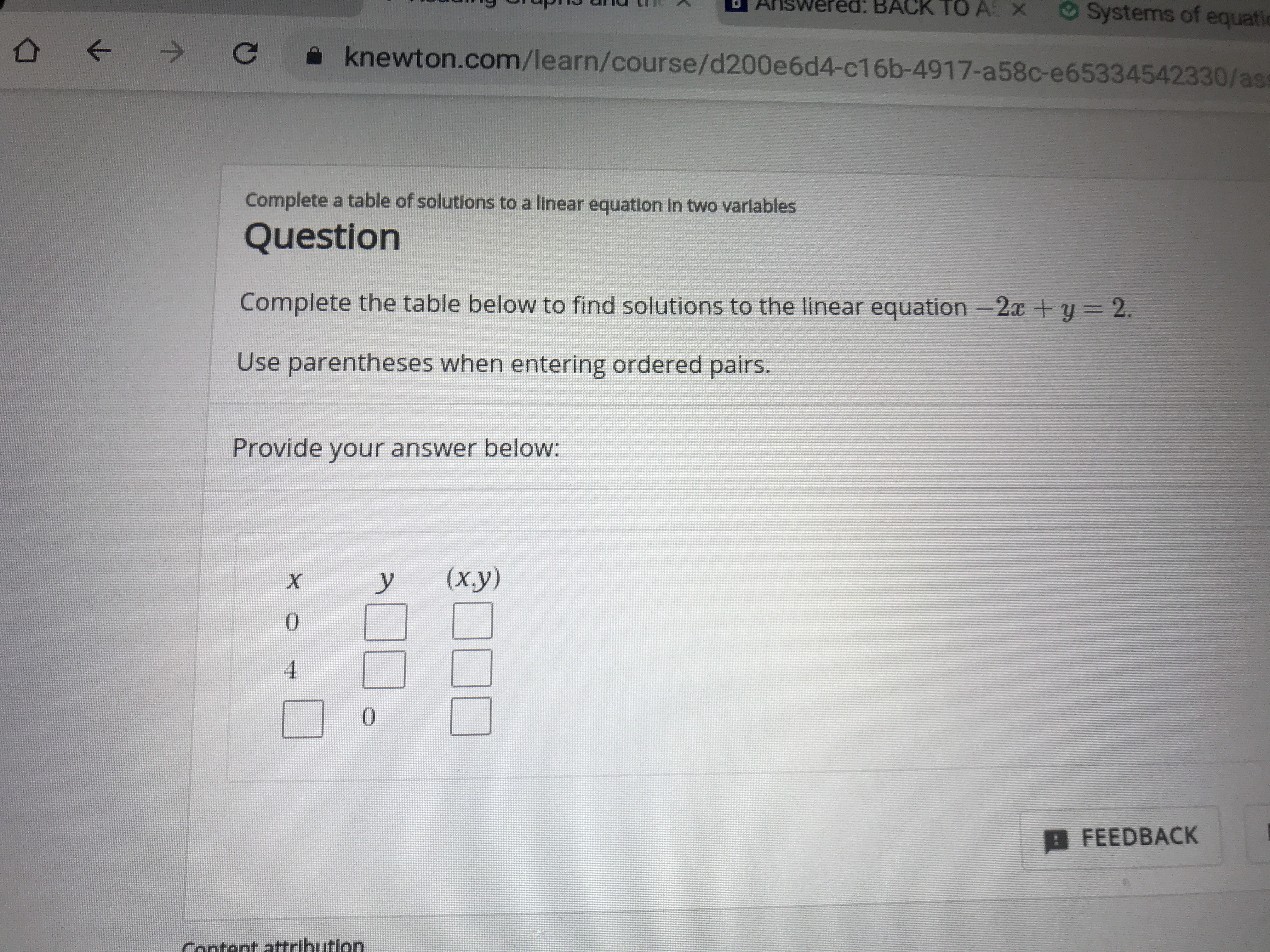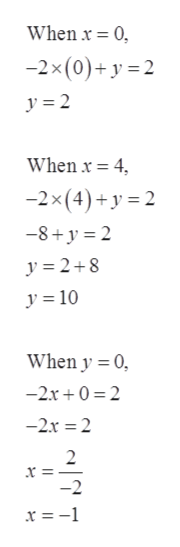# Systems of equatiBACK TO AS Xknewton.com/learn/course/d200e6d4-c16b-4917-a58c-e65334542330/asComplete a table of solutions to a linear equation in two variablesQuestionComplete the table below to find solutions to the linear equation -2x +y= 2.Use parentheses when entering ordered pairs.Provide your answer below:(ху)У040B FEEDBACKContent attribution

Questionhelp_outlineImage TranscriptioncloseSystems of equati BACK TO AS X knewton.com/learn/course/d200e6d4-c16b-4917-a58c-e65334542330/as Complete a table of solutions to a linear equation in two variables Question Complete the table below to find solutions to the linear equation -2x +y= 2. Use parentheses when entering ordered pairs. Provide your answer below: (ху) У 0 4 0 B FEEDBACK Content attribution fullscreen
check_circle

Step 1

Given:

Step 2

Now according to the question, ...help_outlineImage TranscriptioncloseWhen x 0 -2x (0)+y 2 y 2 When x = 4 -2x (4)+y 2 -8+y 2 y 2+8 y 10 When y 0 -2r0 2 -2x 2 2 x = -2 x = -1 fullscreen

### Want to see the full answer?

See Solution

#### Want to see this answer and more?

Solutions are written by subject experts who are available 24/7. Questions are typically answered within 1 hour.*

See Solution
*Response times may vary by subject and question.
Tagged in

### Equations and In-equations